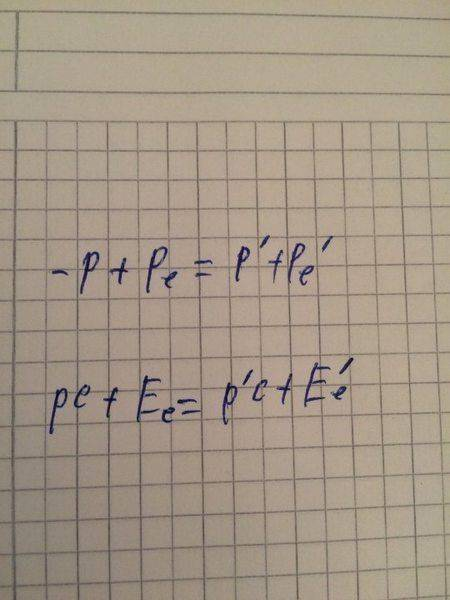# Photon colliding with moving electron

## Homework Statement

Consider Comton scattering of a photon by a moving electron. Before the collision the photon has wave length λ and is moving in the +x direction, and the electron is moving in the -x direction with total energy E. The photon and electron collide head-on. After the collision both are moving in the -x direction. (a) Derive an expression for the wavelength λ' of the scattered photon. (b) Show that for the case E>>mc^2, the result reduces to λ′= hc/E(1+ m^2 c^4 λ/4hcE)

## The Attempt at a Solution

I tried using energy and momentum conservation laws but can't seem to find it

I would but i haven't done much progress
I just need a hint or the direction of the solution

Conserving momentum and energy should get you to the answer, put in the vectors correctly while conserving momentum.

Conserving momentum and energy should get you to the answer, put in the vectors correctly while conserving momentum.
did that
thx anyway

Einitial=Efinal
pinitial=pfinal
Plug in the values, get an answer, post the equations and somebody will point out what was wrong in them.

•Giorgi1997
Einitial=Efinal
pinitial=pfinal
Plug in the values, get an answer, post the equations and somebody will point out what was wrong in them.This is how i try to start